### Home > PC > Chapter 8 > Lesson 8.2.6 > Problem8-152

8-152.
1. Copy the steps below and fill in the blanks to prove that n ! > 2n for n ≥ 4. Note that we do not start with n = 1. Verify that the statement is false for n < 4. Homework Help ✎

1. Verify the statement is true for n = 4.

2. Assume the statement is true for n = k.

3. Prove the statement is true for n = k + 1.

Recall that: (k + 1)! = ____ (k + 1)

We assumed that k ! > 2k , therefore (k + 1)! = ____ (k + 1) > ____ (k + 1).

Because k ≥ 4, k + 1 > 2 and hence ____ (k + 1) > 2k · ____ = 2−.

Following the steps from above, we have shown that ____ > ____.

4. Write a conclusion for your proof by induction.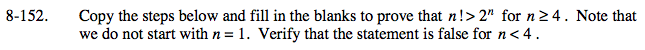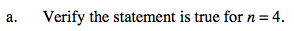4! = 24 > 24 = 16 whereas 3! = 6 ≯ 23 = 8.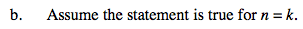Assume that k! > 2k for k ≥ 4.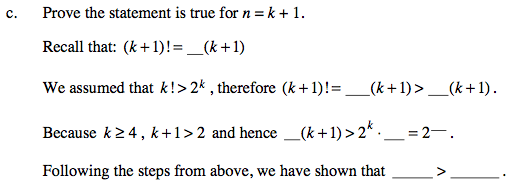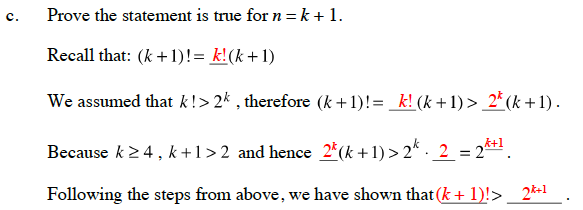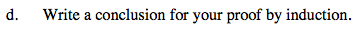Hence, by mathematical induction we have proven that n! > 2n for n ≥ 4.